# Python的运算符

...PythonPython大约 3 分钟

### # 算数运算符号

+加号，连接符号
-减号
*乘号
/除号
%除法的余数
//除法商的整数部分
**乘方

``````print(3+2)
``````

``````print("3+2")
``````

True正确
False错误

### # 比较符号

>大于号
>=大于或等于号
==等于号
!=不等于号
<小于号
<=小于或等于号

• `=`为赋值号，如`a=1`定义`a=1`（人类理解的等于号）

• `==`为等于号（Python理解的等于号），如`a==1`表示`a=1`（人类理解的等于号）

or
and
not表否定
in在...内
is是（相当于==）

### # 赋值号

=赋值c=a
+=加法赋值c=a+b
-=减法赋值c=a-b
*=乘法赋值c=a*b
/=除法赋值c=a/b
%=余数赋值c=a%b
//=商赋值c=a//b
**乘方赋值c=a**b

``````a = 1 # 赋值
a += 1 # 加法赋值
print(a) # 输出 a 的值
``````

### # 位运算符

&按位与两个的二进制都为1才是1，否则为0

^按位异或两个的二进制都相等则为0，否则为1

``````3&2 # 输出 2

3|2 # 输出 3

3^2 # 输出 1
``````

### # 区别

• `=`为赋值号，如`a=1`即定义a=1（人类理解的等于号）；`==`为等于号（Python理解的等于号），如`a==1`表示a=1（人类理解的等于号）

• `is`用于判断两个变量的引用是否为同一个内存地址（可使用`id（）`查看）；`==` 用于判断两个变量的值是否相等。

### # 特别注意

1. 整除运算符`//`是向下取整，而取整函数`int()`是向零取整。
2. 对于正数的取余与数学上的取余没有区别，但是对于负数，Python里取余后要取绝对值。
3. 布尔非运算符的输出结果永远是True或者False，不像布尔与运算符和布尔或运算符。

•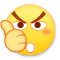0
•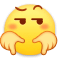0
•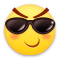0
•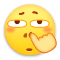0
•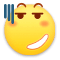0
•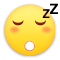0

• 按正序
• 按倒序
• 按热度